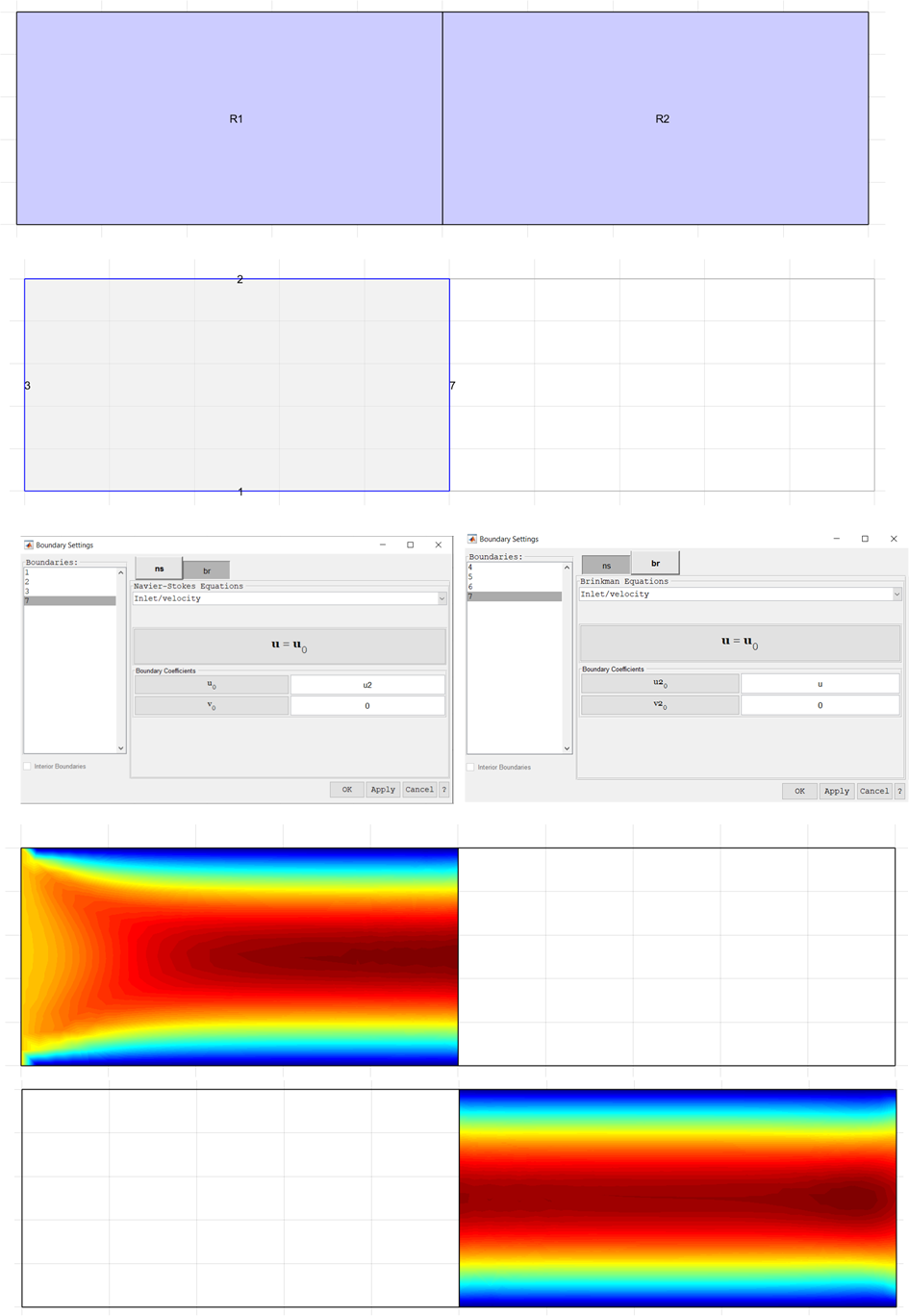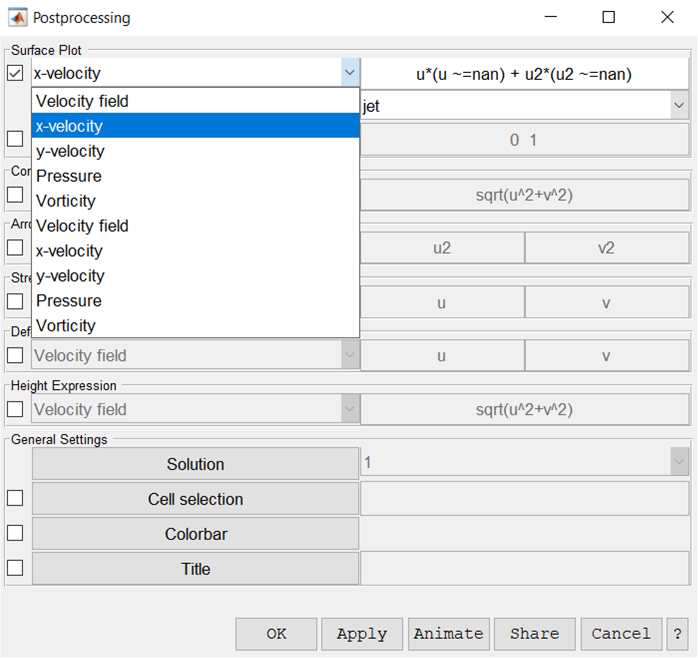# Navier-Stokes and Brinkman EquationsClassicListThreaded4 messagesOpen this post in threaded view
|

## Navier-Stokes and Brinkman Equations

 Hi, I am trying to use Navier-Stokes and Brinkman Equations for the flow in porous media (see the test domain below, where R2 is the porous media, and flow is from R1 to R2). I followed your example (https://www.featool.com/doc/multiphysics_02_porous_flow1#tut_mph02) to build the model, but I have several questions: (1) I set the boundary between R1 and R2 according to your example, i.e. for R1 - inlet velocity u2 and for R2 inlet velocity u. For more complicated test domain, I am wondering if there will be flow from R2 to R1 if the boundary is set up as inlet velocity for both domain? Actually I did find the backward flow arrows in other more complicated test domains. Here I just used a simple example. (2) In the Brinkman equations, why there is no parameter for porosity? Perhaps it is lumped into other parameters? It will be great if porosity can be set up by users. (3) Would it be possible to show the surface plots for both R1 and R2 simultaneously? Now it can only be shown separately for R1 and R2 for the flow if I choose x-velocity. Thank you for your help,Open this post in threaded view
|

## Re: Navier-Stokes and Brinkman Equations

 Administrator (1) I set the boundary between R1 and R2 according to your example, i.e. for R1 - inlet velocity u2 and for R2 inlet velocity u. For more complicated test domain, I am wondering if there will be flow from R2 to R1 if the boundary is set up as inlet velocity for both domain? Actually I did find the backward flow arrows in other more complicated test domains. Here I just used a simple example. The boundary conditions will only specify the the flow (velocity values) from one domain is equal to the other. The direction and magnitudes can not be influenced by these internal conditions, and are entirely due to those of your other boundaries and prescribed conditions. (2) In the Brinkman equations, why there is no parameter for porosity? Perhaps it is lumped into other parameters? It will be great if porosity can be set up by users. You would have to add this manually be editing the equation (using the "edit" button in the subdomain settings dialog box). (3) Would it be possible to show the surface plots for both R1 and R2 simultaneously? Now it can only be shown separately for R1 and R2 for the flow if I choose x-velocity. You can enter and plot a custom combined expression, for example:  u*(u~=nan) + u2*(u2~=nan) the solution variables take nan (not a number) values in non active domains, and can be "filtered" using logical switch expressions (which evaluate to 1 or 0) as above.
 In reply to this post by James Thank you for your answers. I tried your plot solution to show both u and u2 simultaneously. Unfortunately, it did not work (see image below). In the expression setup, it seems the first x-velocity is for u, and the second x-velocity is for u2. If I chose the first x-velocity, the expression setup did not recognize u2, although there were no error messages. In addition, I found that in the plot expression field, u~=nan (an example you gave in the answer) will cause an error but u ~=nan (with a space between u and ~) can pass the OK/apply button. Thank you for your consideration!Administrator I see, yes apparently everything multiplied with "nan" will give nan so this approach will not work. You should be able to use the "setnan" function instead like described in step 86. of the porous media flow tutorial: https://www.featool.com/doc/multiphysics_02_porous_flow1https://www.featool.com/doc/setnan_8m.html setnan(sqrt(u^2+w^2)) + setnan(sqrt(u2^2+w2^2))